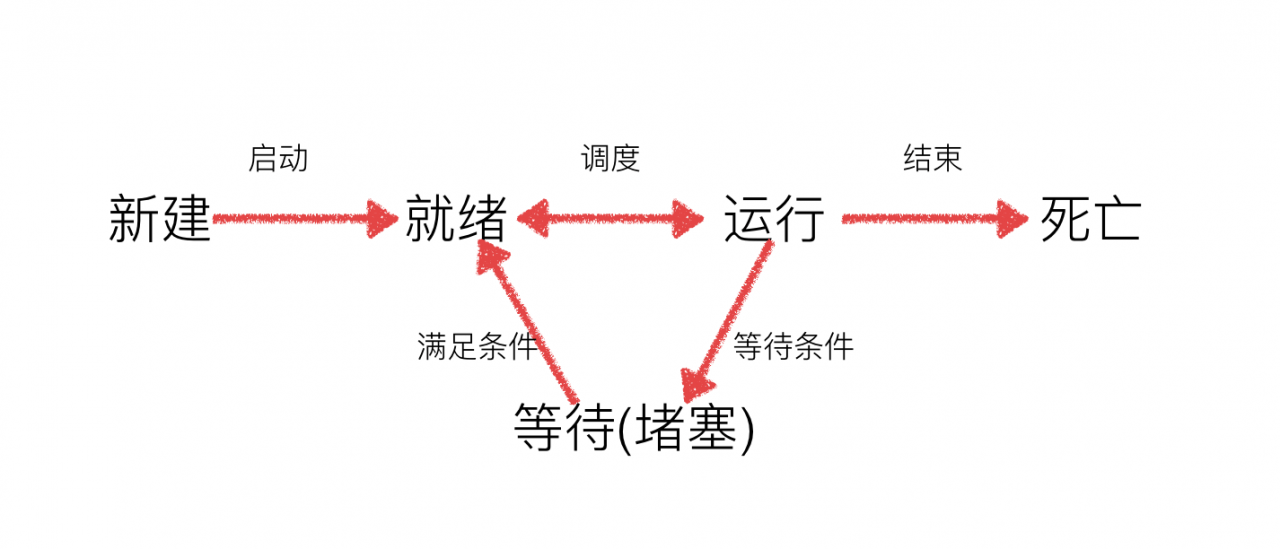533次阅读```#coding=utf-8
import time
def run(self):
for i in range(3):
time.sleep(1)
msg = "I'm "+self.name+' @ '+str(i) #name属性中保存的是当前线程的名字
print(msg)
def run(self):
for i in range(3):
time.sleep(1)
msg = "I'm "+self.name+' @ '+str(i) #name属性中保存的是当前线程的名字
print(msg)
if __name__ == '__main__':
t1.start()
t2.start()```2. 线程的执行顺序

```#coding=utf-8
import time
def run(self):
for i in range(3):
time.sleep(1)
msg = "I'm "+self.name+' @ '+str(i) #name属性中保存的是当前线程的名字
print(msg)
def test():
for i in range(1,5):
t.start()
if __name__ == '__main__':
test()```